# Electromagnetically Induced Transparency and Atomic Polarization

An atomic system consisting of two lower states connected to one upper state by two coherent light fields (a "Λ system") can exhibit the phenomenon known as electromagnetically induced transparency (EIT), discussed in the example "Three-level System: Electromagnetically Induced Transparency". Such a system can take the form of the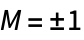levels of aground state connected to aexcited state by two light beams with opposite circular polarizations (and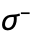). If the frequency of one light field (the "pump") is held fixed while the frequency of the other (the "probe") is varied, a drop in absorption of the probe beam is seen when the probe frequency matches that of the pump. This EIT resonance is associated with coherence between the two ground-state levels, which in the case considered here can be interpreted as angular-momentum polarization of theground state.

In this example we calculate the EIT signal measured on atransition, and show how the EIT resonance can be understood in terms of the ground-state polarization, which we illustrate using population plots and angular-momentum probability surfaces (see "Angular-momentum Probability Surfaces").

## Solve the system

We first define the atomic system and form the Hamiltonian. We will perform the calculation in a frame in which the Hamiltonian is time independent, and there is a steady-state solution to the Bloch equations. Thus it is convenient to write the density-matrix elements without explicit time dependence.

 Set the option TimeDependence for the DensityMatrix function.
 Define the atomic system.

The total optical field consists of the sum of the pump field:light (ellipticity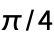) with frequency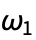and "reduced" Rabi frequency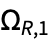, and the probe field: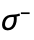light (ellipticity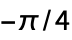) with frequency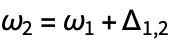and reduced Rabi frequency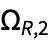.

 Define the total optical field.
 The Hamiltonian for the system subject to the combined light field.
 The level diagram for the system and the Hamiltonian, showing a resonant pump field and detuned probe field.

We then apply the rotating-wave approximation, going into the frame "rotating" at the pump frequency, and writingin terms of its detuning from resonance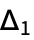. In this frame, the Hamiltonian has time dependence at the difference of the two light frequencies,.

 Apply the rotating-wave approximation, removing all frequencies in the optical range from the Hamiltonian.

In performing the RWA, we have used a unitary transformation with a term oscillating at the pump frequency.

 The unitary transformation used for the rotating-wave approximation.

The remaining time dependence can be removed by making an additional unitary transformation, with a term oscillating at the difference frequency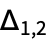.

 Define a unitary matrix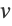with a term oscillating at the difference frequency.
 Transform the Hamiltonian into the frame specified by.

Note that no terms are dropped in this step; this is just a change of basis, not a further approximation.

In addition to the Hamiltonian, terms describing relaxation and repopulation are needed to form the Liouville equation. The relaxation matrix describes transit relaxation (atoms leaving the light beam) at a rate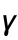and spontaneous decay of the upper state at the specified rate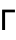.

 Form the relaxation matrix.

The repopulation matrix describes transit repopulation (atoms entering the light beam) and repopulation due to atoms that have decayed from the upper state.

 Form the repopulation matrix.

We can now form and solve the Liouville equation (Bloch equations) under the steady-state condition.

 The evolution equations.

In order to speed up the computation, we specialize to the case of equal-intensity light beams and pump light on resonance.

## Absorption signal

In a classic EIT experiment, the pump frequency is held fixed, the probe frequency is swept, and the absorption of the probe light is measured. We can plot this absorption spectrum using the solution we have found. We consider here the case of equal-intensity pump and probe beams, because the resulting population and angular-momentum probability plots more clearly illustrate the effect in this case. When equal-intensity beams are used, the effect is sometimes referred to as coherent population trapping (CPT), in order to distinguish it from the classic EIT case of a strong pump and weak probe, but the physical mechanism of the effect is identical in the two cases.

The function Observables takes the transition frequency, light electric field, and light polarization as arguments and returns the change induced in the various light polarization parameters due to interaction with the atoms, in terms of the rotating-frame density-matrix elements. Here we use the probe frequency. We also need to specify the transform from the stationary frame that we have used to eliminate time dependence in the density matrix; the total transform is given by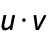.

 The absorption of the probe in terms of the density-matrix elements.
 Substitute the density matrix into the expression for the absorption.

We can simplify the expression for absorption by writing the Rabi frequency in terms of the saturation parameter, and making the assumption that the transit relaxation rate is much slower than the excited-state decay rate: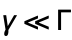.

 Apply a replacement rule that neglects γ compared to Γ.
 A list of experimental parameters that we will use for the plots.
 Plot the absorption.

We see a classic EIT/CPT absorption spectrum. There are three regimes:

(1) When the probe detuning is much larger than the power-broadened natural width, the probe is off resonance and there is little absorption.

(2) When the probe detuning is less than the power-broadened natural width, but greater than the power-broadened ground-state transit width, there is absorption with a spectrum that is qualitatively the same as if there were no pump field.

(3) When the pump-probe detuning is within the power-broadened ground-state transit width, a sharp EIT resonance is seen: the absorption is dramatically reduced.

## Zeeman populations

In order to understand the EIT spectrum, we can plot the atomic density matrix in different ways. One way is to display the populations of the Zeeman sublevels.

 Write the solution for the density matrix in terms of κ.
 Make an interactive diagram showing the level structure, the light fields, and the population of each Zeeman sublevel as the probe frequency is changed.

As the probe frequency is swept through the resonance, we see three different behaviors corresponding to the three regimes discussed above:

(1) When the probe is far off resonance, it has little effect on the atoms. The redistribution of the ground-state populations is primarily due to the pump light: it pumps out the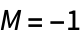sublevel, and these atoms are redistributed evenly between theand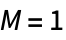sublevels. The populations of these two sublevels are not exactly equal because of the residual effect of the detuned probe light. Since the probe is off resonance, it experiences little absorption.

(2) When the probe detuning is within the natural width, the probe optically pumps the atoms, in addition to the pump. In this regime, the pump and probe act incoherently: the pump pumps out thesublevel and the probe pumps out thesublevel; the atoms are transferred to thesublevel. As the probe pumps thesublevel, it is absorbed: this accounts for the increase of absorption in this regime.

(3) When the pump-probe detuning is within the ground-state transit width, the pump and probe act coherently: they do not act independently on theandsublevels, but rather work together to pump out a "bright state" consisting of a linear superposition of the two sublevels. Atoms remain in the "dark state" consisting of the orthogonal linear superposition, so that theandsublevels are not completely depleted. Since the pump and probe both act on the same (bright) state, the bright state is very efficiently pumped, leaving fewer atoms to interact with the probe light. Thus the absorption of the probe drops in this regime.

## The light polarization

In order to understand why the pump and probe act incoherently in regime (2) but coherently in regime (3), we can look at the polarization of the total light field consisting of the pump plus the probe. The pump and probe have the same amplitude but opposite circular polarizations, so that the combination of the two is a linearly polarized light field. However, since the pump and probe have different frequencies, there is a constantly changing phase between them. This means that the direction of the resultant linear polarization constantly changes. Here we make an interactive animation of the light polarization as a function of time.

First we find the polarization of the total light field. The optical frequency of this field is the average of the pump and probe frequencies; we divide out the time dependence at this frequency so that only the time dependence of the light polarization remains. When we take the real part, we find the direction of the total light electric field amplitude as a function of time.

 Find the polarization direction of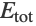as a function of time.
 Make an interactive plot that animates the light polarization direction for a given pump-probe detuning.

We see that the light polarization rotates at a rate given by the detuning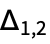. Only when the pump and probe frequencies are equal is the polarization direction fixed. This gives the distinction between regimes (2) and (3):

Since the result of optically pumping the ground state is effectively averaged over the ground-state relaxation time, when the polarization direction makes a complete revolution during this time, the light is seen by the atoms as unpolarized (it has equal polarization in every direction perpendicular to the light propagation direction). Unpolarized light acts incoherently on the different states that it interacts with. Thus, in regime (2), where the light polarization rotates rapidly compared to the ground-state relaxation time, the two light fields act independently on the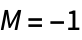and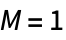sublevels.

On the other hand, in regime (3), the light polarization rotates slowly compared to the ground-state relaxation time. This means that the atoms, rather than seeing two circularly polarized pump and probe fields, effectively see light with linear polarization along some direction perpendicular to the light propagation direction. Such polarization does not pump on an eigenstate of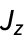, but rather on a superposition of eigenstates, namely the bright state discussed above.

## Cartesian populations

We can see this from another perspective by plotting the populations of the sublevels, not in the Zeeman basis, but rather in the basis of the dark and bright states. Note that these states depend on the direction of the light polarization, so that in the lab frame they change continuously for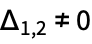. By making the unitary transformation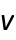above, we have performed the calculation in a rotating frame in which the bright and dark states are fixed and correspond to theandstates in the Cartesian basis. Thestate is the same as thestate in the Zeeman basis with the quantization axis along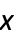, while thestate is the corresponding state with the quantization axis along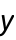. These states, along with thestate in the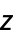basis, make a complete set of sublevels for astate, and the density matrix can be written in terms of these sublevels.

Note that, because of the finite ground-state relaxation time, the dark and bright states can not, in a certain sense, "keep up" with the light polarization if it is rotating very quickly, due to the averaging effect discussed above. Thus the light effectively interacts with both the dark and bright states for detuned pump and probe. Even though the dark and bright states are not deserving of their names in this case, they are still well defined as certain linear combinations of the Zeeman sublevels, and we can track their populations.

We can illustrate the averaging effect for the light by finding the effective optical pumping strengths of the light on the dark and bright states and drawing darker or lighter arrows corresponding to these strengths. We can find the pumping strengths by taking the time-dependent Hamiltonian in the lab-frame Cartesian basis and averaging it over time with a exponentially decaying weighting factor corresponding to the ground-state-polarization decay rate.

 Transform the density matrix and Hamiltonian into the Cartesian basis.
 Find effective pumping strengths by integrating the Hamiltonian over time with a weighting factor.
 Make an interactive diagram for the rotating-frame Cartesian basis. The arrows showing the light-induced coupling have Opacity given by the effective pumping strength found above.

The three ground-state sublevels now correspond to thestate (identical to the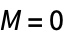sublevel) and the dark and bright linear combinations of theand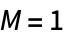sublevels. For, the pump and probe fields interact with both the dark and bright states, as can be seen on the diagram. We can examine the three regimes discussed above in terms of this diagram:

(1) When the probe is far detuned, only the pump beam is effective. Since it interacts equally with both the dark and bright states, these states are equally pumped into thestate, which is the only true dark state in this regime.

(2) As the probe is tuned onto optical resonance, it also becomes effective, pumping more atoms into the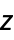state. Since the pump and probe act incoherently in this regime (the resultant light polarization rotates too quickly for the atomic polarization to "keep up"), both the dark and bright states are pumped equally.

(3) When the probe frequency is tuned close enough to that of the pump, the pump and probe fields add coherently. The combination of the pump and probe field that interacts with the bright state is reinforced, while the combination that interacts with the dark state cancels, as seen in the diagram. Thus the bright state is pumped efficiently into the dark andstates. Since the probe light only probes the population of the bright state, the absorption of the probe drops in this regime.

## Angular-momentum probability surfaces

The limitation of the population plots, as we can see, is that they are very different depending on the chosen basis; in addition, the plot for any given basis does not contain complete information about the system. There is an alternative method for displaying the ground-state polarization that is basis-independent and contains the complete information about the ground-state density matrix. This is the method of angular-momentum probability surfaces (see "Angular-momentum Probability Surfaces").

Here we plot the angular-momentum probability surfaces for the ground-state polarization in each of the three regimes.

 The ground-state density matrix.
 Angular-momentum probability surfaces for regimes (1), (2), and (3).

The above discussion can be largely summed up in terms of these plots.